# 7.4 Boolean Algebraic Properties

### The Commutative Property

Another type of mathematical identity, called a “property” or a “law,” describes how differing variables relate to each other in a system of numbers. One of these properties is known as the commutative property, and it applies equally to addition and multiplication. In essence, the commutative property tells us we can reverse the order of variables that are either added together or multiplied together without changing the truth of the expression: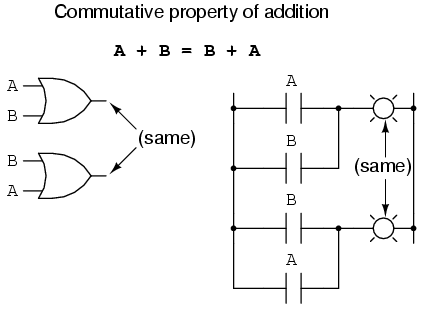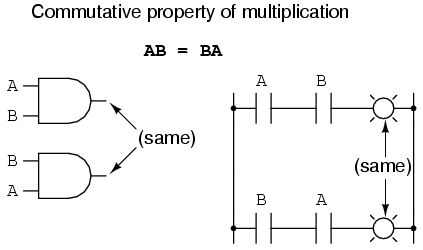### The Associative Property

Along with the commutative properties of addition and multiplication, we have the associative property, again applying equally well to addition and multiplication. This property tells us we can associate groups of added or multiplied variables together with parentheses without altering the truth of the equations.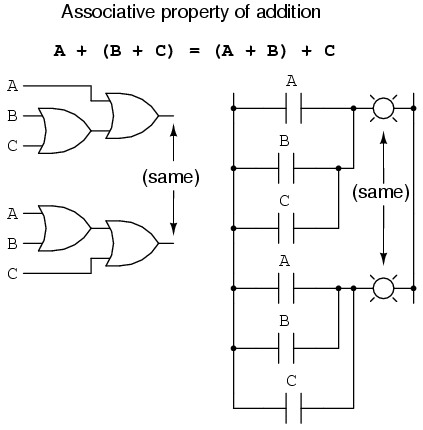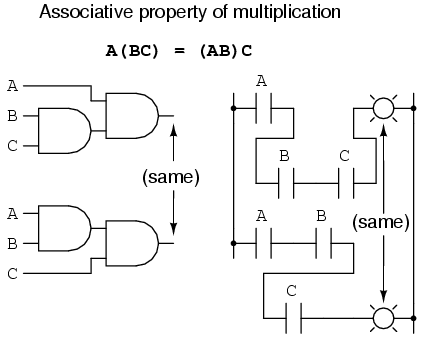### The Distributive Property

Lastly, we have the distributive property, illustrating how to expand a Boolean expression formed by the product of a sum, and in reverse shows us how terms may be factored out of Boolean sums-of-products: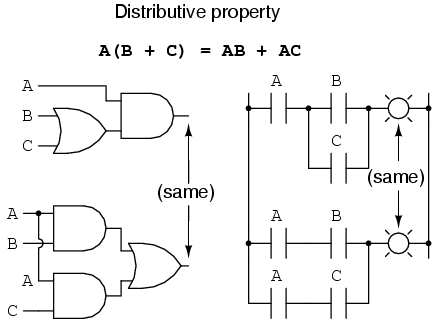To summarize, here are the three basic properties: commutative, associative, and distributive.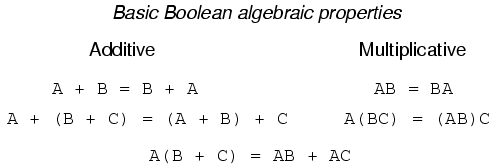Back to Main Index of Book